# Operations and equations with directed number

### Term 2 starting in week 7 :: Estimated time: 3 weeks

• Understand and use representations of directed numbers
• Order directed numbers using lines and appropriate symbols
• Perform calculations that cross zero
• Subtract directed numbers
• Multiplication of directed numbers
• Multiplication and division of directed numbers
• Use a calculator for directed number calculations
• Evaluate algebraic expressions with directed number
• Introduction to two-step equations
• Solve two-step equations
• Use order of operations with directed numbers

For higher-attaining pupils:

• Roots of positive numbers
• Explore higher powers and roots

This page should remember your ticks from one visit to the next for a period of time. It does this by using Local Storage so the information is saved only on the computer you are working on right now.

## Lesson Starters

Here are some suggestions for whole-class, projectable resources which can be used at the beginnings of each lesson in this block.

### 1st Lesson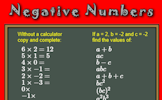#### Negative Numbers

Perform calculations involving negative numbers

### 2nd Lesson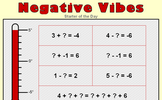#### Negative Vibes

Practise techniques for answering questions involving negative numbers.

### 3rd Lesson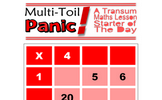#### Multi-Toil Panic

Copy and complete the multiplication grid. The higher levels include negative numbers.

### 4th Lesson### 5th Lesson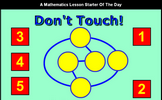#### Don't Touch!

A number placing puzzle which, when solved, raises the notion of proof.

### 6th Lesson#### Clouds

Some calculations are partly obscured. Work out what those calculations might be.

### 7th Lesson#### For Starters

Four problems involving addition, subtraction, multiplication and division

### 8th Lesson### 9th Lesson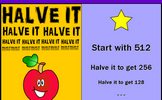#### Halve it

Start with 512. Halve it to get 256. Halve it to get 128. Continue as far as possible.

Some of the Starters above are to reinforce concepts learnt, others are to introduce new ideas while others are on unrelated topics designed for retrieval practice or and opportunity to develop problem-solving skills.

White Rose ResourcesEnd of block assessments provide a quick progress check at the end of each block of learning to make sure students have understood the content covered. This Scheme of Learning was produced by White Rose Maths and is used here with permission granted on 30th June 2021.For All: